# Trivia Questions

Popular Maths Questions over the years!### Primary 3 & 4

Primary 3 Question 1Primary 3 Question 2Primary 3 Question 3Primary 4 Question 1Primary 4 Question 2Primary 4 Question 3Primary 4 Question 4### Primary 5 & 6

Assumption Method - Type 3Grouping Concepts 2 - Type 4Simultaneous Equation (Replacement) - Type 2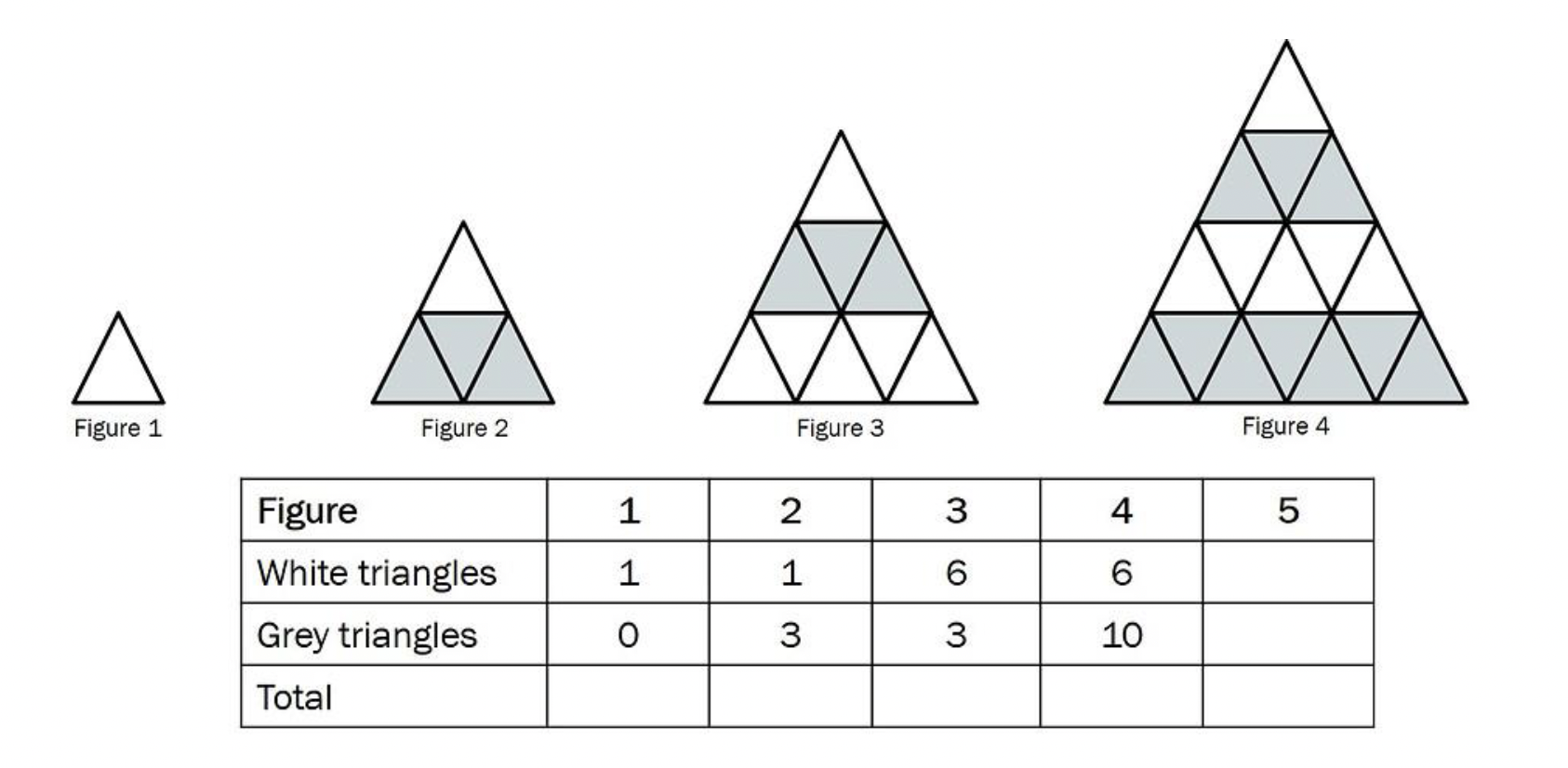(a) Fill in the table above. (1m)
(b) Find the total number of grey and white triangles for Figure 250. (1m)
(c) Find the percentage of grey triangles in Figure 250. (3m)

(a)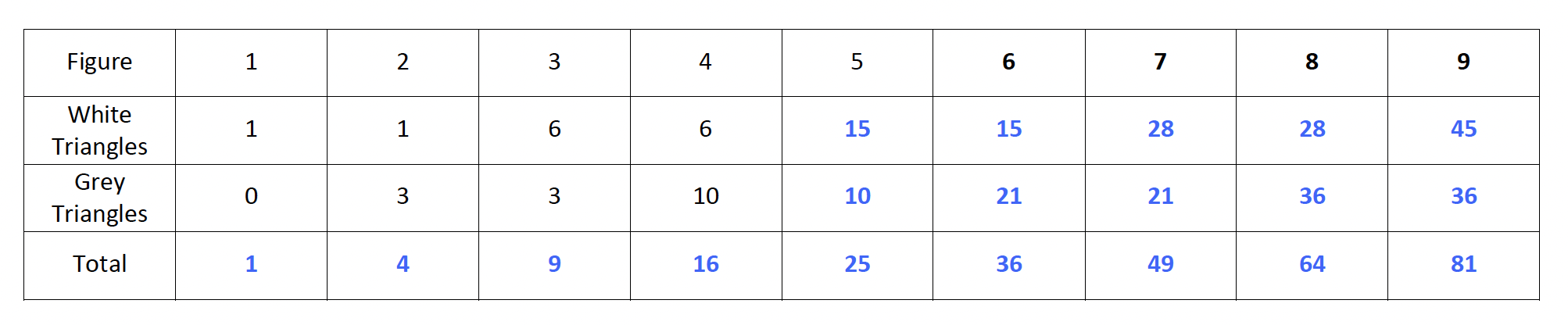(b) The total number of triangles is just figure number -> n x n

Figure 250 -> 250 x 250 = 62 500

(c) Let’s extend the table in (a).

From (a), notice a pattern!

In order to find the number of grey triangles, we use this formula -> (N÷2) x (N+1)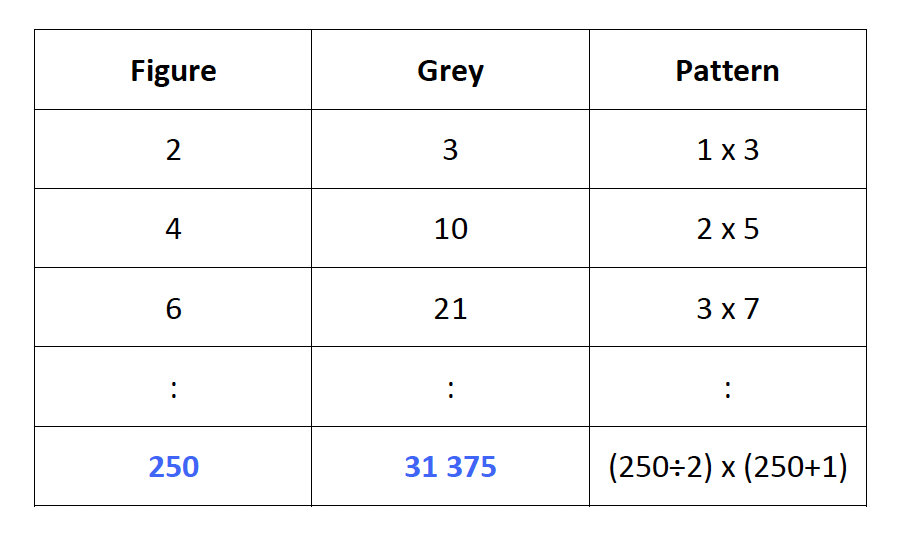Percentage of grey triangles in Figure 250: 31 375 ÷ 62 500 x 100% = 50.2%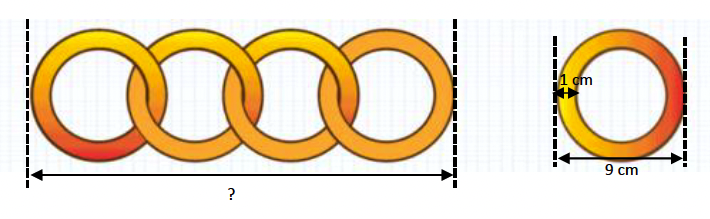4 similar golden rings overlap each other as shown below. Each ring has a diameter of 9 cm and a thickness of 1 cm.

(a) Find the length of the 4 overlapping rings.
(b) If the length of the first ring to the last ring is 198 cm, how many rings are there?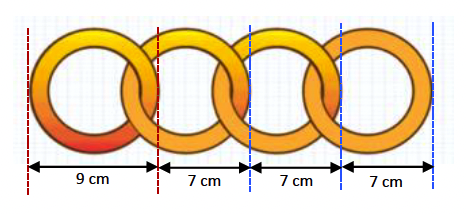(a) 9 + (3 x 7) = 30 cm The length of 4 overlapping rings is 30 cm.

(b) Only the first ring is 9 cm while the other length of the rings is of the same length.

198 cm – 9 cm = 189 cm
189 cm ÷ 7 = 27
Number of rings -> 27 + 1 = 28

There are 28 rings.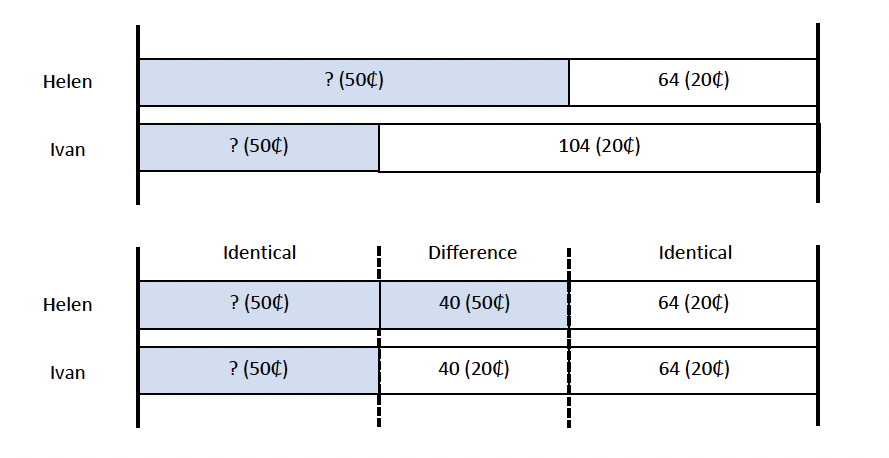Helen and Ivan had the same number of coins.
Helen had a number of 50-cent coins and 64 20-cent coins. Helen’s coins had a total mass of 1.134 kg. Ivan had a number of 50-cent coins and 104 20-cent coins.

(a) Who has more money in coins and by how much more?
(b) Given that each 50-cent coin is 2.7g heavier than a 20-cent coin, what is the total mass of Ivan’s coins in kilograms?

(a) Helen has more money because she has more 50-cent coins by the amount 40 x (50₵ -20₵) = 1200₵ = \$12

Each 50₵ coin is 2.7g heavier than a 20₵ coin, Mass of Helen’s coins – Mass of Ivan’s coins = Difference in Total Mass 40 x 2.7 g = 108 g = 0.108 kg

Mass of Helen’s coins -> 1.134 kg Mass of Ivan’s coins -> 1.134 kg – 0.108 kg = 1.026 kg

(b) The total mass of Ivan’s coins is 1.026 kg.

### Secondary 1 & 2

Secondary 1Secondary 2### Secondary 3 & 4

Secondary 3 E-MathSecondary 4 E-MathSecondary 3 A-MathSecondary 4 A-Math